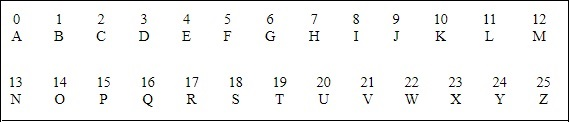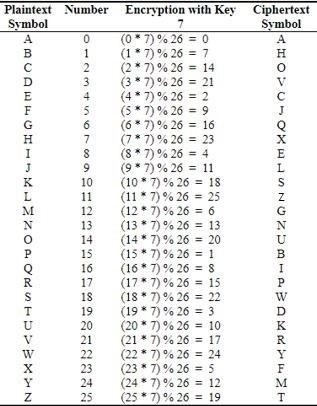# Multiplicative Cipher

While using Caesar cipher technique, encrypting and decrypting symbols involves converting the values into numbers with a simple basic procedure of addition or subtraction.

If multiplication is used to convert to cipher text, it is called a wrap-around situation. Consider the letters and the associated numbers to be used as shown below −The numbers will be used for multiplication procedure and the associated key is 7. The basic formula to be used in such a scenario to generate a multiplicative cipher is as follows −

(Alphabet Number * key)mod(total number of alphabets)


The number fetched through output is mapped in the table mentioned above and the corresponding letter is taken as the encrypted letter.The basic modulation function of a multiplicative cipher in Python is as follows −

def unshift(key, ch):
offset = ord(ch) - ASC_A
return chr(((key * (offset + key)) % WIDTH) + ASC_A)


Note − The advantage with a multiplicative cipher is that it can work with very large keys like 8,953,851. It would take quite a long time for a computer to brute-force through a majority of nine million keys.